# High School Math : How to find the length of the diagonal of a rectangle

## Example Questions

### Example Question #1 : How To Find The Length Of The Diagonal Of A Rectangle

What is the diagonal of a rectangle with a side length of 5 and a base of 8? (Round to the nearest tenth)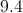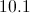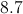Explanation:

To find the diagonal of a rectangle we must use the side lengths to create a 90 degree triangle with side lengths of 5, 8, and a hypotenuse which is equal to the diagonal.

If we have a right angle triangle and a value for two of the three side lengths, we use the Pythagorean Theorem to solve for the length of the third side.

The two side lengths that meet to form the right angle are labeledandwhich are interchangeable for each side length.

The long length connecting them is labeledand is known as the hypotenuse.

The Pythagorean Theorem states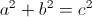Take 5 and 8 and plug them into the equation asandto yield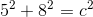First square the numbers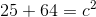After squaring the numbers add them together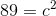Once you have the sum, square root both sides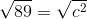After calculating our answer for the diagonal is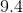.

### Example Question #21 : Quadrilaterals

What is the length of the diagonal of a rectangle that is 3 feet long and 4 feet wide?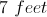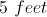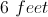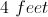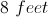Explanation:

The diagonal of the rectangle is equivalent to finding the length of the hypotenuse of a right triangle with sides 3 and 4. Using the Pythagorean Theorem: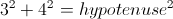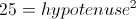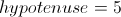Therefore the diagonal of the rectangle is 5 feet.

### Example Question #1 : How To Find The Length Of The Diagonal Of A Rectangle

The length and width of a rectangle are in the ratio of 3:4. If the rectangle has an area of 108 square centimeters, what is the length of the diagonal?

15 centimeters

12 centimeters

24 centimeters

9 centimeters

18 centimeters

15 centimeters

Explanation:

The length and width of the rectangle are in a ratio of 3:4, so the sides can be written as 3x and 4x.

We also know the area, so we write an equation and solve for x:

(3x)(4x) = 12x= 108.

x2 = 9

x = 3

Now we can recalculate the length and the width:

length = 3x = 3(3) = 9 centimeters

width = 4x = 4(3) = 12 centimeters

Using the Pythagorean Theorem we can find the diagonal, c:

length2 + width2 = c2

92 + 12= c2

81 + 144 = c2

225 = c2

= 15 centimeters

### All High School Math Resources